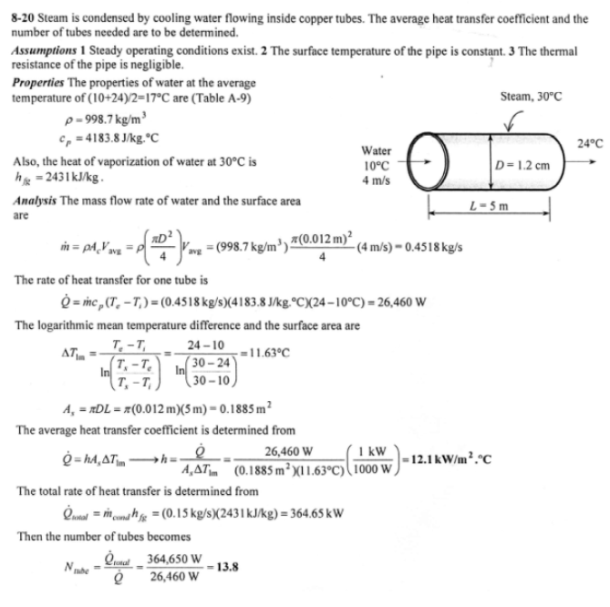heat and mass transfer problems engineering equations heat and mass transfer school homework engineering heat and mass transfer formulas heat and mass transfer solutions to heat and mass transfer problems full solution engineering problem solution heat and mass transfer math problems engineering equations heat and mass transfer school homework engineering solutions to heat and mass transfer formulas heat problems mass problem solutions to transfer problems full solution heat and mass transfer
heat and mass transfer problems engineering equations heat and mass transfer school homework engineering heat and mass transfer formulas heat and mass transfer solutions to heat and mass transfer problems full solution engineering problem solution heat and mass transfer math problems engineering equations heat and mass transfer school homework engineering solutions to heat and mass transfer formulas heat problems mass problem solutions to transfer problems full solution heat and mass transfer
Highalphabet Home Page heat and mass transfer problem solutions Heat and Mass Transfer Page
Cooling water available at 10 C is used to condense steam at 30 C in the condenser of a power plant at a rate of 0.15 kg/s by circulating the cooling water through a bank of 5-m-long 1.2-cm-internal-diameter thin copper tubes. Water enters the tubes at a mean velocity of 4 m/s and leaves at a temperature of 24 C. The tubes are nearly isothermal at 30 C. Determine the average heat transfer coefficient between the water, the tubes, and the number of tubes needed to achieve the indicated heat transfer rate in the condenser.Cooling water available at 10 C is used to condense steam at 30 C in the condenser of a power plant at a rate of 0.15 kg/s by circulating the cooling water through a bank of 5-m-long 1.2-cm-internal-diameter thin copper tubes. Water enters the tubes at a mean velocity of 4 m/s and leaves at a temperature of 24 C. The tubes are nearly isothermal at 30 C. Determine the average heat transfer coefficient between the water, the tubes, and the number of tubes needed to achieve the indicated heat transfer rate in the condenser.